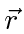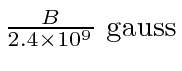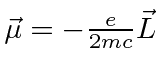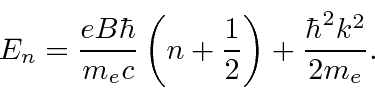## The Quantum Hamiltonian Including a B-field

We will quantize the Hamiltonianin the usual way, by replacing the momentum by the momentum operator, for the case of a constant magnetic field.

Note that the momentum operator will now include momentum in the field, not just the particle's momentum. As this Hamiltonian is written,is the variable conjugate toand is related to the velocity byas seen in our derivation of the Lorentz force.

The computation yieldsThe usual kinetic energy term, the first term on the left side, has been recovered. The standard potential energy of an electron in an Electric field is visible on the right side. We see two additional terms due to the magnetic field. An estimate of the size of the two B field terms for atoms shows that, for realizable magnetic fields, the first term is fairly small (down by a factor ofcompared to hydrogen binding energy), and the second can be neglected. The second term may be important in very high magnetic fields like those produced near neutron stars or if distance scales are larger than in atoms like in a plasma (see example below).

So, for atoms, the dominant additional term is the one we anticipated classically in section 18.4,where. This is, effectively, the magnetic moment due to the electron's orbital angular momentum. In atoms, this term gives rise to the Zeeman effect: otherwise degenerate atomic states split in energy when a magnetic field is applied. Note that the electron spin which is not included here also contributes to the splitting and will be studied later.

The Zeeman effect, neglecting electron spin, is particularly simple to calculate because the the hydrogen energy eigenstates are also eigenstates of the additional term in the Hamiltonian. Hence, the correction can be calculated exactly and easily.

* Example: Splitting of orbital angular momentum states in a B field.*

The result is that the shifts in the eigen-energies arewhereis the usual quantum number for the z component of orbital angular momentum. The Zeeman splitting of Hydrogen states, with spin included, was a powerful tool in understanding Quantum Physics and we will discuss it in detail in chapter 23.

The additional magnetic field terms are important in a plasma because the typical radii can be much bigger than in an atom. A plasma is composed of ions and electrons, together to make a (usually) electrically neutral mix. The charged particles are essentially free to move in the plasma. If we apply an external magnetic field, we have a quantum mechanics problem to solve. On earth, we use plasmas in magnetic fields for many things, including nuclear fusion reactors. Most regions of space contain plasmas and magnetic fields.

In the example below, we will solve the Quantum Mechanics problem two ways: one using our new Hamiltonian with B field terms, and the other writing the Hamiltonian in terms of A. The first one will exploit both rotational symmetry about the B field direction and translational symmetry along the B field direction. We will turn the radial equation into the equation we solved for Hydrogen. In the second solution, we will use translational symmetry along the B field direction as well as translational symmetry transverse to the B field. We will now turn the remaining 1D part of the Schrödinger equation into the 1D harmonic oscillator equation, showing that the two problems we have solved analytically are actually related to each other!

* Example: A neutral plasma in a constant magnetic field.*

The result in either solution for the eigen-energies can be written aswhich depends on 2 quantum numbers.is the conserved momentum along the field direction which can take on any value.is an integer dealing with the state in x and y. In the first solution we understandin terms of the radial wavefunction in cylindrical coordinates and the angular momentum about the field direction. In the second solution, the physical meaning is less clear.

Jim Branson 2013-04-22